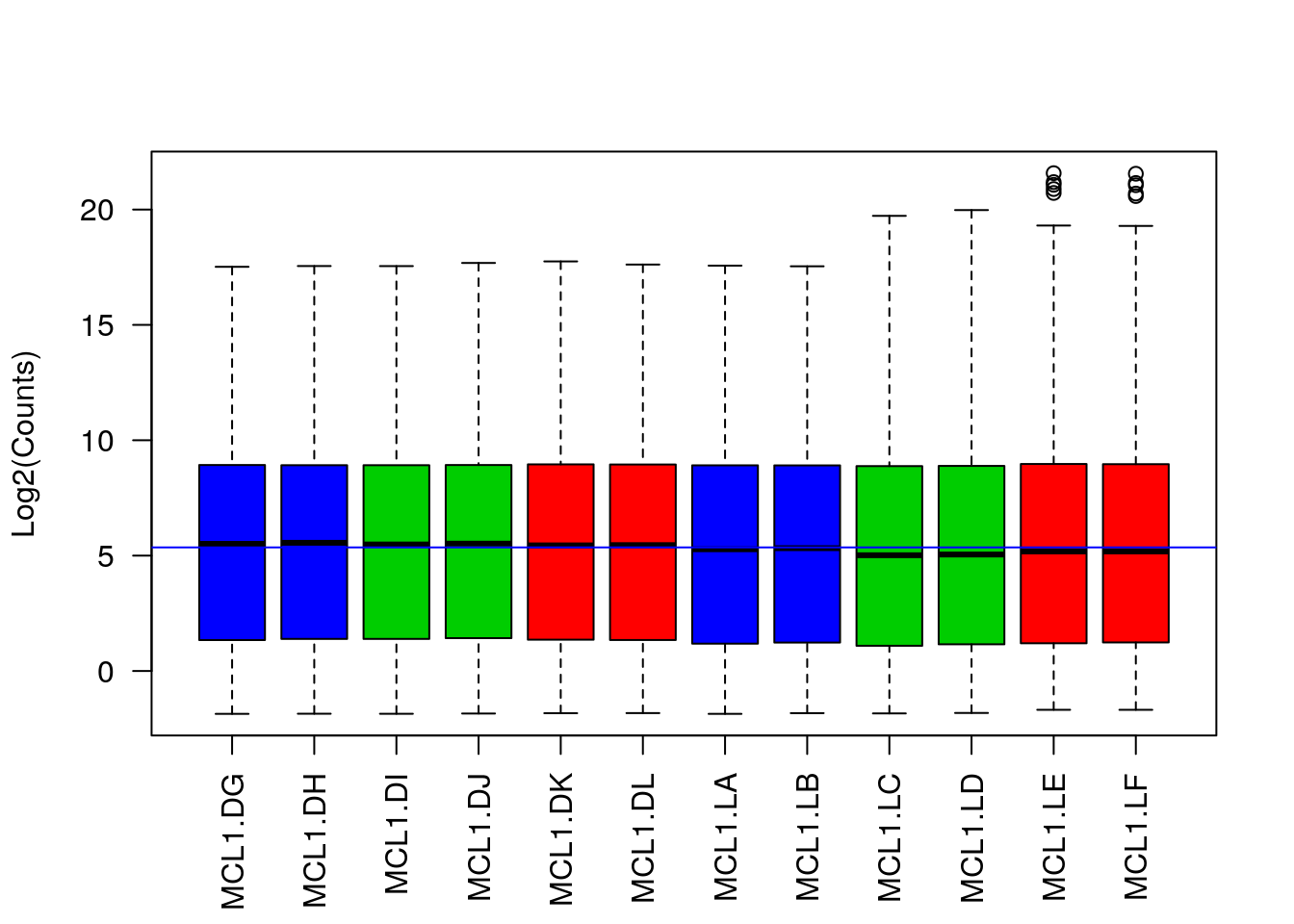Data

library(DESeq2)
library(gplots)
library(RColorBrewer)
library(limma)
library(tidyverse)

Challenge 1

1. Use the DESeq2 function rlog to transform the count data. This function also normalises for library size.
2. Plot the count distribution boxplots with this data How has this effected the count distributions?
rlogcounts <- rlog(countdata)

statusCol <- as.numeric(factor(sampleinfo\$Status)) + 1
# Check distributions of samples using boxplots
boxplot(rlogcounts,
xlab="",
ylab="Log2(Counts)",
las=2,
col=statusCol)
# Let's add a blue horizontal line that corresponds to the median logCPM
abline(h=median(as.matrix(logcounts)), col="blue")Challenge 2

Plot the biased and unbiased MA plots both samples side by side to see the before and after normalisation.

par(mfrow=c(2,2))
plotMA(logcounts, array = 7, main="MCL1.LA - Raw")
abline(h=0,col="grey")
plotMA(logNormalizedCounts, array = 7, main="MCL1.LA - Normalised")
abline(h=0,col="grey")
plotMA(logcounts, array = 11, main="MCL1.LE - Raw")
abline(h=0,col="grey")
plotMA(logNormalizedCounts, array = 11, main="MCL1.LE - Normalised")
abline(h=0,col="grey")